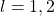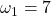The post has two goals:

(1) Explain how to forecast volatility using a simple Heterogeneous Auto-Regressive (HAR) model. (Corsi, 2002)
(2) Check if higher moments like Skewness and Kurtosis add forecast value to this model.

It will be a high frequency analysis as the data is recorded on minutely basis. The purpose is to construct an accurate proxy for the daily volatility using this data, and forecast ahead using the HAR model.

Heterogeneous Auto-Regressive (HAR) model
The model is created to capture few stylized facts observed in the data. Among others, volatility distribution has fat tails, meaning we observe very high or very low values with relatively high probability. Also, volatility has long memory, so a value ‘long ago’, for example, 20 days ago, may still have an impact on the value we will see tomorrow. We can estimate a very long Auto-regressive model, say 30 lags, but that might lead to over fitting and apart from that, it may not be necessary to allow this kind of flexibility. The model itself is very simple:where,. We can useand, corresponding with one week and one month. That way, we do not need to estimate so many lags.

Win: No need to estimate 30 lags.
lose: Flexibility. We assume a constant influence of all lags in the same “time zone”.

For example, lagged volatility of the order 2 through 7 will have same coefficient. The “…” is there to remind you that you can add more variables that you think can help in prediction. For example, we will add lagged return and the lagged sign of the return, which will add asymmetry effect, like in GJR-Garch or Asymmetric garch models.

In sum, it is a very simple linear regression.

Data for this tutorial can be found here. After saving the data you can load it as follows:

?View Code RSPLUS
 load(file = ".../prlev2.RData")

What is loaded is an array named prlev with dimension 390 – 8 – 250 which is 390 – minutes of 8 – (high close, etc.. as in Yahoo.finance) for the 250 – days ending in 22/10/2012. The ticker is SPY.

In order to construct the model we want to create the‘s. I use the intra-day prices and construct what is called the Realiized Range over a time interval of one day. One estimator is the Garman-Klass. Few more can be found here.

?View Code RSPLUS
 GarmanKlass = function(x){ # x is an array of prices with dimension: # (num.intervals.each.day)*( (5 or 6), "open", "high" etc)*(number.days) n <- dim(x) # number of intervals each day. l <- dim(x) # number of days gk = NULL for (i in 1:l) { log.hi.lo <- log( x[1:n,2,i]/x[1:n,3,i] ) log.cl.to.cl <- log( x[2:n,4,i]/x[1:(n-1),4,i] ) gk[i] = ( sum(.5*log.hi.lo^2) - sum( (2*log(2) - 1)*(log.cl.to.cl^2) ) ) /n } return(gk) } volat = sqrt(GarmanKlass(prlev))

So now volat is a vector of 250 observations, each represents the realized volatility for each day.

Next we construct the right hand side which will help to clarify the model above:

?View Code RSPLUS
 library(moments) # for skewness and kurtosis calculation n = dim(prlev) ctc = (prlev[390,4,2:n]-prlev[390,4,1:(n-1)])/prlev[390,4,1:(n-1)]#Create Close to close returns ## Make room for whatever you want to use for forecasting - right hand side variables volatw = NULL volatm = NULL volatd = NULL lagret0 = NULL lagsign = NULL lagkurtosis = NULL lagskewness = NULL ## k0 = apply(prlev[,1,],2,kurtosis) ## Maybe Kurtosis is important s0 = apply(prlev[,1,],2,skewness) ## Maybe skewness is important TT = length(volat) for (i in 30:TT){ volatd[i] = volat[(i-1)] # this is sigma_{t-1} # Not exactly as in the equation above but equivalent: volatw[i] = sum(volat[(i-7):(i-2)],na.rm = T)/6 volatm[i] = sum(volat[(i-29):(i-8)],na.rm = T)/22 lagret0[i] = ret0[(i-1)] # lagged returns lagsign[i] = sign(ret0[(i-1)]) # lagged sign returns lagkurtosis[i] = k0[(i-1)] # lagged Kurtosis lagskewness[i] = s0[(i-1)] # lagged skewness }

This is pretty much all the hard work we have for today. Now it is a matter of simple lm function.

?View Code RSPLUS
 har0 = lm(volat~volatd+volatw+volatm+lagret0+lagsign ) ;summary(har0)   Coefficients: Estimate Std. Error t value Pr(>|t|) (Intercept) 1.99e-04 1.23e-05 16.19 < 2e-16 *** volatd 1.65e+02 7.91e+01 2.09 0.03807 * volatw 5.78e+02 1.51e+02 3.84 0.00016 *** volatm 2.43e+02 1.16e+02 2.09 0.03826 * lagret0 -1.70e-03 1.01e-03 -1.69 0.09220 . lagsign -1.29e-05 8.63e-06 -1.49 0.13729 --- Signif. codes: 0 '***' 0.001 '**' 0.01 '*' 0.05 '.' 0.1 ' ' 1   Residual standard error: 8.21e-05 on 211 degrees of freedom (33 observations deleted due to missingness) Multiple R-squared: 0.353, Adjusted R-squared: 0.338 F-statistic: 23 on 5 and 211 DF, p-value: <2e-16

So, we see the effect of volatility clustering, the volatility today, the volatility of past week and past month all have significant impact on the volatility tomorrow. Positive sign means that when they are up, the volatility today, on average, goes up as well.

It might be interesting to see if higher moments help to predict, so let’s incorporate the lagged (intra-day) skewness and kurtosis we collected before.

?View Code RSPLUS
 har1 = lm(volat~volatd+volatw+volatm+lagret0+lagsign+ lagkurtosis + lagskewness) ; summary(har1)   Coefficients: Estimate Std. Error t value Pr(>|t|) (Intercept) 1.55e-08 1.27e-08 1.22 0.22478 volatd 7.16e-02 7.88e-02 0.91 0.36482 volatw 5.12e-01 1.50e-01 3.42 0.00076 *** volatm 2.09e-01 1.14e-01 1.83 0.06829 . lagret0 -1.45e-06 1.02e-06 -1.42 0.15617 lagsign -1.15e-08 8.53e-09 -1.35 0.17838 lagkurtosis -6.73e-09 5.74e-09 -1.17 0.24274 lagskewness -1.15e-08 9.40e-09 -1.23 0.22182 --- Signif. codes: 0 '***' 0.001 '**' 0.01 '*' 0.05 '.' 0.1 ' ' 1   Residual standard error: 8.02e-08 on 209 degrees of freedom (33 observations deleted due to missingness) Multiple R-squared: 0.257, Adjusted R-squared: 0.233 F-statistic: 10.3 on 7 and 209 DF, p-value: 3.93e-11

No, they are not important, that is, once you account for the lagged volatility and allow for asymmetry in the form of lagsign, there is not much added value in the higher moment. At least not prediction value.

References

here is a working paper version of the HAR paper by Corsi (2002).

# Never miss an update! Subscribe to R-bloggers to receive e-mails with the latest R posts.(You will not see this message again.)

Click here to close (This popup will not appear again)